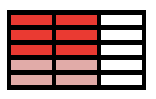### Home > CC1MN > Chapter 6 > Lesson 6.3.1 > Problem6-72

6-72.

Zac is making cookies, but he does not have enough brown sugar to make a full recipe. The full recipe calls for $\frac { 2 } { 3 }$ cup of brown sugar.

If Zac has enough brown sugar for $\frac { 3 } { 5 }$ of the full recipe, how much brown sugar does he have?

1. Represent the $\frac { 2 } { 3 }$ cup of brown sugar the recipe calls for with a diagram.

First draw a rectangle to represent a full cup of brown sugar. Now try to solve the problem with this information.

$\frac{2}{3} \text{ is the same thing as 2 one-thirds.}$

2. Represent the portion of brown sugar that Zac has if he makes only $\frac { 3 } { 5 }$ of the recipe.

How can you subdivide your shaded diagram from part (a) into fifths (5 equal parts)?

Which part of the sugar is 3 of the fifths?

One way this can be represented is...Label the portion of brown sugar Zac needs.

3. What mathematical operation should Zac use to find the amount of brown sugar he has? Write an expression and then calculate its value.

• $\text{What fraction of a cup will Zac use? What operation with } \frac{2}{3} \text{ and } \frac{3}{5} \text{ will get that answer?}$